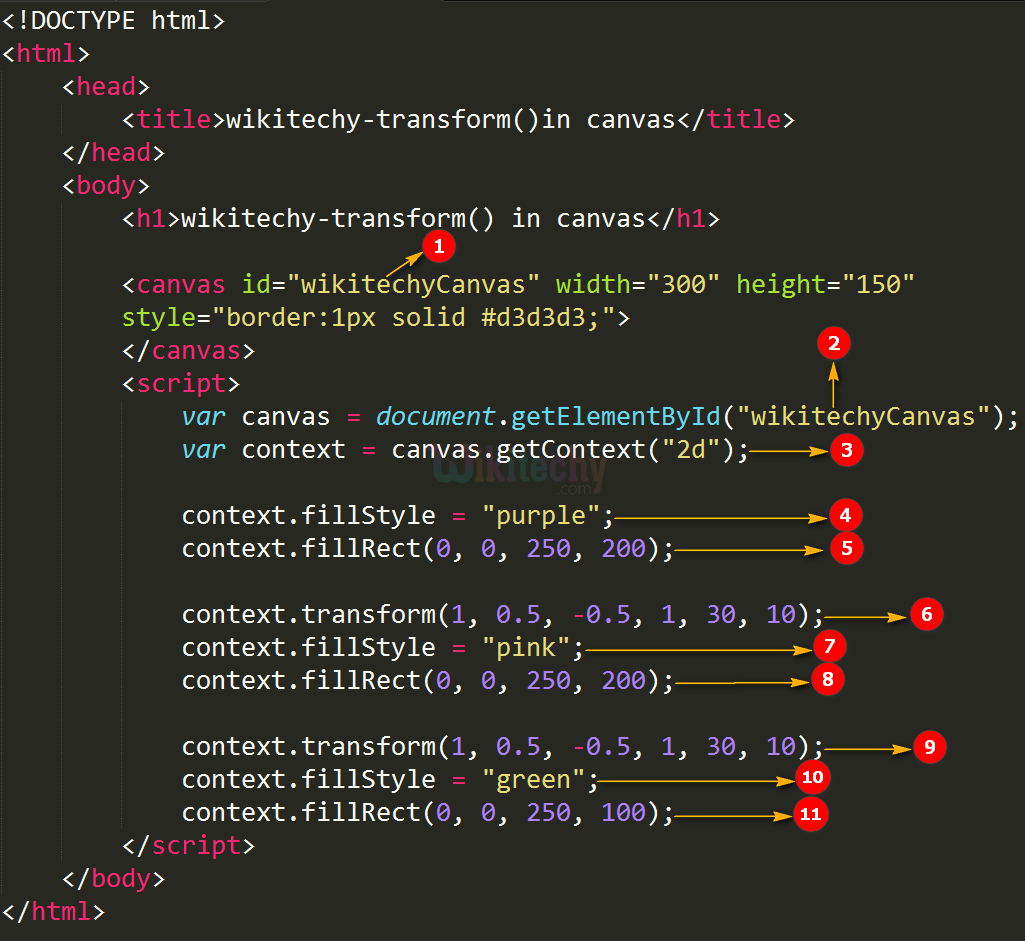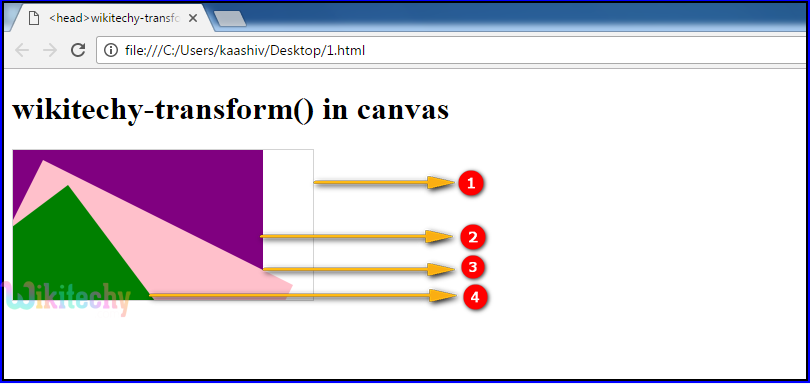# html tutorial - transform() Method in HTML5 Canvas - html5 - html code - html formLearn html - html tutorial - Transform method in html5 canvas - html examples - html programs

• The transform() is the method of HTML CANVAS.
• The transform() method is used to transform the current diagram by using the transformation matrix like an identity matrix.
• The transform() method is used to scale, rotate, move, and skew the diagram.

## Parameter values for transform() Method in HTML5 Canvas:

Parameter Description
a Horizontal scaling
b Horizontal skewing
c Vertical skewing
d Vertical scaling
e Horizontal moving
f Vertical moving

## Code Explanation for transform() Method in HTML5 Canvas:1. ”wikitechyCanvas” is used to declare the id value of the canvas tag.
2. The getElementById() method is used to get the element with the specific id (“wikitechyCanvas”).
3. canvas.getContext(“2d”) method returns an object that provides methods and properties for drawing on the canvas.
4. The context.fillStyle() method is used to fillStyle of a diagram (purple color rectangular box).
5. The context.fillRect() method is used to draws a "filled" rectangle (0,0,250,200) in (x0,y0,x1,y1).
6. The context.transform() method is used to “transform” a diagram (1, 0.5, -0.5, 1, 30, 10) in (a,b,c,d,e,f).
7. The context.fillstyle() method is used to fillstyle of a diagram (pink color rectangular box).
8. The context.fillRect() method is used to draws a "filled" rectangle (0,0,250,200) (x0,y0,x1,y1).
9. The context.transform() method is used to “transform” a diagram (1, 0.5, -0.5, 1, 30, 10) (a,b,c,d,e,f).
10. The context.fillstyle() method is used to fillstyle of a diagram (green color rectangular box).
11. The context.fillRect() method is used to draws a "filled" rectangle (0, 0, 250, 100) in (x0,y0,x1,y1).

## Output for transform() Method in HTML5 Canvas:1. <canvas> tag is used to draw a rectangle with gray border.
2. The rectangle is drawn with this parameter (0,0,250,200) and filled with purple color
3. The rectangle is drawn with this parameter (0,0,250,250)and filled with pink color
-context.transform(1, 0.5, -0.5, 1, 30, 10);
4. The rectangle is drawn with this parameter (0,0,250,100)and filled with green color
-context.transform(1, 0.5, -0.5, 1, 30, 10);

## Browser Support for transform() Method in HTML5 Canvas:Yes 9.0 Yes Yes Yes

## Tips and Notes

• The setTransform() method does not behave relatively to other transformations.